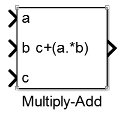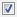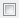## Library

HDL Coder / HDL Operations

•## Description

The Multiply-Add block computes the product of the first two inputs, a and b, and adds the result to the third input, c. The inputs can be vectors or scalars.

The multiplication operation is full precision, regardless of the output type. The Integer rounding mode, Output data type, and Saturate on integer overflow settings apply only to the addition operation.

Use the Multiply-Add block to map a combined multiply-add or a multiply-subtract operation to a DSP unit in your target hardware. You can select the Function setting in the Block Parameters dialog box for the Multiply-Add block.

To map to a DSP unit, specify the `SynthesisTool` property for your model. When you generate HDL code for your model, HDL Coder™ configures the multiply-add operation so that your synthesis tool can map to a DSP unit.

Note

Some DSP units do not have the multiply-add capability. To see if your hardware has the multiply-add capability, refer to the documentation for the hardware.

## Data Type Support

The Multiply-Add block accepts and outputs signals of numeric data type that Simulink® supports, including fixed-point data types.

You can use matrix data types with the Multiply-Add block. When you use these types, the port dimensions of the inputs `a` and `b` must match. For example, in MATLAB®, you can perform these matrix operations:

```a = [1 2; 3 4]; b = [5; 6]; c = 7; c + (a.*b)```
```ans = 12 17 25 31 ```
However, when you perform this multiplication in the Simulink environment, you see an error: ```Error in port widths or dimensions```.

## Parameters

### Function

Specify the function to perform a combined multiply and add or a multiply and subtract operation.

Settings

Default: `c+(a.*b)`

You can set the function to:

• `c+(a.*b)`

• `c-(a.*b)`

• `(a.*b)-c`

### Output data type

Specify the output data type.

Settings

Default: `Inherit: Inherit via internal rule`

Set the output data type to:

• A rule that inherits a data type, for example, ```Inherit: Same as input```

• An expression that evaluates to a valid data type, for example, `fixdt([],16,0)`

Click the buttonto display the Data Type Assistant dialog box, which helps you to set the Output data type parameter.

### Integer rounding mode

Specify the rounding mode for fixed-point operations.

Settings

Default: `Floor`

`Ceiling`

Rounds positive and negative numbers toward positive infinity. Equivalent to the MATLAB `ceil` function.

`Convergent`

Rounds number to the nearest representable value. If a tie occurs, rounds to the nearest even integer. Equivalent to the Fixed-Point Designer™ `convergent` function.

`Floor`

Rounds positive and negative numbers toward negative infinity. Equivalent to the MATLAB `floor` function.

`Nearest`

Rounds number to the nearest representable value. If a tie occurs, rounds toward positive infinity. Equivalent to the Fixed-Point Designer `nearest` function.

`Round`

Rounds number to the nearest representable value. If a tie occurs, rounds positive numbers toward positive infinity and rounds negative numbers toward negative infinity. Equivalent to the Fixed-Point Designer `round` function.

`Simplest`

Chooses between rounding toward floor and rounding toward zero to generate rounding code that is as efficient as possible.

`Zero`

Rounds number toward zero. Equivalent to the MATLAB `fix` function.

Command-Line Information

See Block-Specific Parameters for the command-line information.

### Saturate on integer overflow

Specify whether overflows saturate.

Settings

Default: OffOn

Overflows saturate to either the minimum or maximum value that the data type can represent.

For example, an overflow associated with a signed 8-bit integer can saturate to -128 or 127.Off

Overflows wrap to the appropriate value that the data type can represent.

For example, the number 130 does not fit in a signed 8-bit integer and wraps to -126.

Tips
• Consider selecting this check box when your model has a possible overflow and you want explicit saturation protection in the generated code.

• Consider clearing this check box when you want to optimize efficiency of your generated code.

Clearing this check box also helps you to avoid overspecifying how a block handles out-of-range signals. For more information, see Troubleshoot Signal Range Errors.

• When you select this check box, saturation applies to every internal operation on the block, not just the output or result.

• In general, the code generation process can detect when overflow is not possible. In this case, the code generator does not produce saturation code.

Command-Line Information
 Parameter: `SaturateOnIntegerOverflow` Type: character vector Value: `'off'` | `'on'` Default: `'off'`

expand all